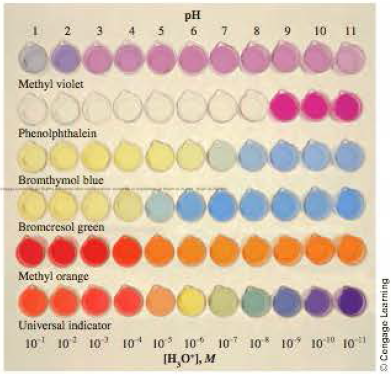# You have solutions of NH 3 , HCl, NaOH, and HC 2 H 3 O 2 (acetic acid), all with the same solute concentrations. Rank these solutions in order of pH, from the highest to the lowest.### General Chemistry - Standalone boo...

11th Edition
Steven D. Gammon + 7 others
Publisher: Cengage Learning
ISBN: 9781305580343### General Chemistry - Standalone boo...

11th Edition
Steven D. Gammon + 7 others
Publisher: Cengage Learning
ISBN: 9781305580343

#### Solutions

Chapter
Section
Chapter 15.8, Problem 15.4CC
Textbook Problem
189 views

## You have solutions of NH3, HCl, NaOH, and HC2H3O2 (acetic acid), all with the same solute concentrations. Rank these solutions in order of pH, from the highest to the lowest.Expert Solution
Interpretation Introduction

Interpretation:

The given solutions in order of pH from the highest to lowest have to be ranked.

Concept introduction:

pH is an logarithmic expression to express a solution is acidic, basic or neutral.  pH scale lies values between 1-14 and on which 7 is neutral, below 7 values are more acidic in nature and above 7 values are more basic in nature.

The pH is equal to pH = -log10c

Where, c is hydrogen ion concentration in moles per liter.

### Explanation of Solution

• From the given solutions, NH3 and NaOH are bases, HCl and HC2H3O2 are acids.
• NaOH is stronger base than NH3 so NaOH solution must have the highest pH value than NH3 solution

### Want to see the full answer?

Check out a sample textbook solution.See solution

### Want to see this answer and more?

Bartleby provides explanations to thousands of textbook problems written by our experts, many with advanced degrees!

See solution